# Segregate even and odd nodes in LinkedList

Problem Statement : Segregate even and odd nodes in LinkedList

Given a LinkedList of integers. Modify the LinkedList in such a way that in Modified LinkedList all the even numbers appear before all the odd numbers in LinkedList.

Also, note that the order of even and odd numbers should remain the same.

Examples:

```Example 1:
Input: 1→2→3→4→5→6→Null
Output: 2→4→6→1→3→5→Null
Explanation :
Odd Nodes in LinkedList are 1,3,5 and
Even Nodes in LinkedList are 2,4,6
In Modified LinkedList all even Nodes comes before
all Odd Nodes. So Modified LinkedList looks like
2→4→6→1→3→5→Null. Order of even and odd Nodes is
maintained in modified LinkedList.

Example 2:
Input: 1→3→5→Null
Output: 1→3→5→Null
Explantion: As there are no Even Nodes in LinkedList,
The Modified LinkedList is same as Original LinkedList.

Example 3:
Input: 2→4→6→8→Null
Output: 2→4→6→8→Null
Explanation: As there are no Odd Nodes in LinkedList,
The Modified LinkedList is same as Original LinkedList.```

Disclaimer: Don’t jump directly to the solution, try it out yourself first.

### Intuition :

The Intuition is to Split the LinkedList into two parts. One Contains all the Even Nodes and the other contains all the Odd Ends. After obtaining two LinkedLists we attach odd LinkedList at the end of the Even LinkedList.

To split the LinkedList take two dummy Nodes which act as the Heads of the odd and Even LinkedList. Traverse the original LinkedList. While traversing if the Node is odd remove the Node from the original LinkedList and add to odd LinkedList. Similarly for the Even Node too.

After Traversing we obtain two LinkedList with all odd and all Even Nodes. Attach odd LinkedList at the end of Even LinkedList. As we are appending nodes to Odd and Even LinkedLists one by one the order of Nodes is undisturbed.

### Algorithm Visual Representation :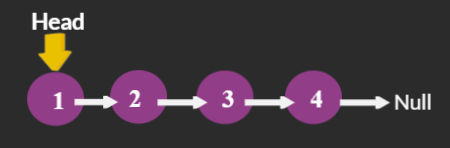Creation of Heads for Even and Odd LinkedList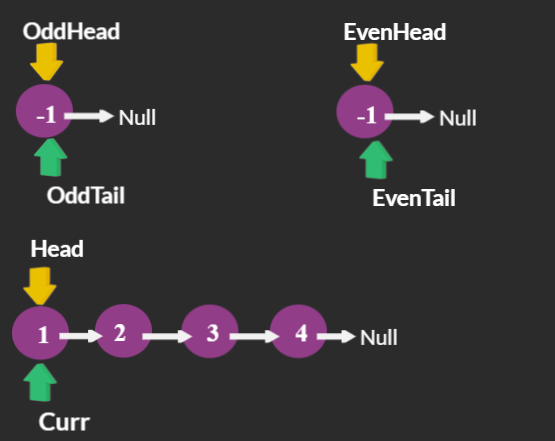As 1 is odd Node, Removing 1 from LinkedList and appending to OddLinkedList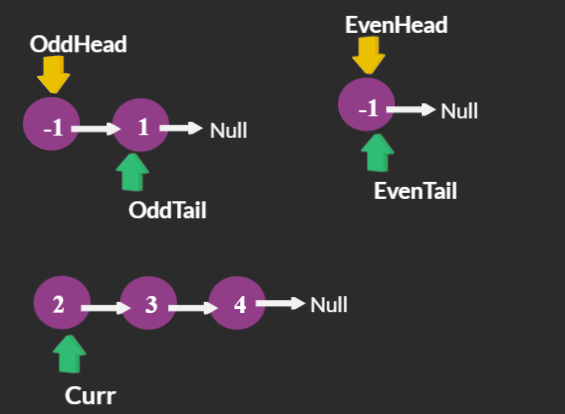As 2 is Even Node, Removing 2 from LinkedList and appending to EvenLinkedList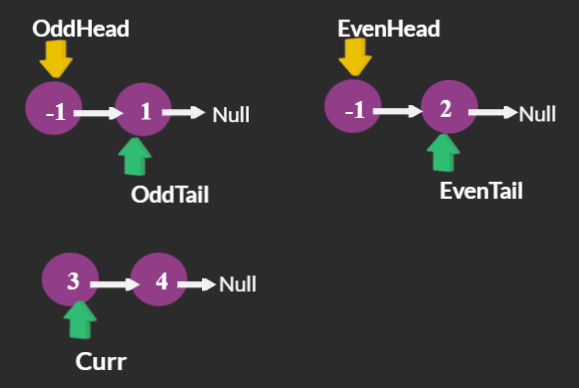As 3 is odd Node, Removing 3 from LinkedList and appending to OddLinkedList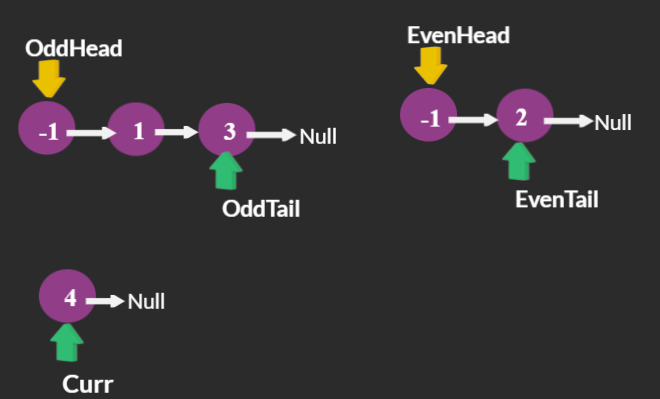As 4 is Even Node, Removing 4 from LinkedList and appending to Even LinkedList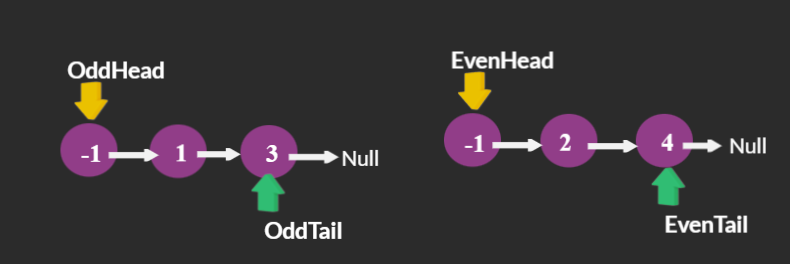Appending OddLinkedList at End of Even LinkedList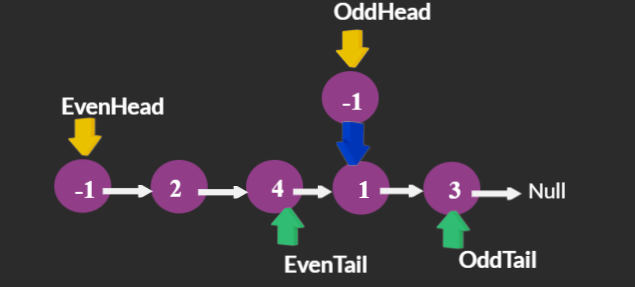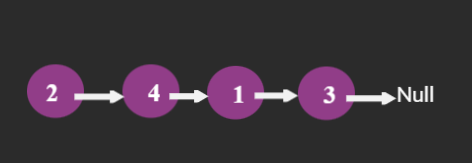Code:

## C++ Code

``````#include <bits/stdc++.h>
using namespace std;

class ListNode
{
public:
int val;
ListNode *next;
ListNode(int x)
{
val = x;
next = nullptr;
}
};

ListNode *head, *tail; // head and tail of the LinkedList

void PrintList(ListNode *head) // Function to print the LinkedList
{
ListNode *curr = head;
for (; curr != nullptr; curr = curr->next)
cout << curr->val << "-->";
cout << "null" << endl;
}

void InsertatLast(int value) // Function for creating a LinkedList
{

ListNode *newnode = new ListNode(value);
if (head == nullptr)
head = newnode, tail = newnode;
else
tail = tail->next = newnode;
}

ListNode *SegregatetoOddEVen()
{
// creating Heads of Odd and Even LinkedLists
ListNode *oddHead = new ListNode(-1), *oddTail = oddHead;
ListNode *evenHead = new ListNode(-1), *evenTail = evenHead;
ListNode *curr = head, *temp;
while (curr)
{
// Breaking the Link of the curr Node.
temp = curr;
curr = curr->next;
temp->next = nullptr;

if (temp->val & 1) // If odd Node,append to odd LinkedList
{
oddTail->next = temp;
oddTail = temp;
}
else // If Even Node,append to even LinkedList
{
evenTail->next = temp;
evenTail = temp;
}
}
// Appending Odd LinkedList at end of EvenLinkedList
}

int main()
{
InsertatLast(1);
InsertatLast(2);
InsertatLast(3);
InsertatLast(4);
cout << "Initial LinkedList : " << endl;
ListNode *newHead = SegregatetoOddEVen();
cout << "LinkedList After Segregration " << endl;
return 0;
}
``````

Output :

1→2→3→4→null

2→4→1→3→null

Time Complexity: O(N) N is the number of Nodes in LinkedList. As we are traversing LinkedList once.

Space Complexity: O(1) We are just Manipulating the Links, not using any extra space.

## Java Code

``````import java.util.*;

class ListNode
{
int val;
ListNode next;
ListNode(int x)
{
val = x;
next = null;
}
}

class TUF{
static ListNode head, tail; // head and tail of the LinkedList

static void PrintList(ListNode head) // Function to print the LinkedList
{
ListNode curr = head;
for (; curr != null; curr = curr.next)
System.out.print(curr.val+"-->");
System.out.println("null");
}

static void InsertatLast(int value) // Function for creating a LinkedList
{

ListNode newnode = new ListNode(value);
if (head == null)
{
tail = newnode;
}
else
tail = tail.next = newnode;
}

static ListNode SegregatetoOddEVen()
{
// creating Heads of Odd and Even LinkedLists
ListNode oddHead = new ListNode(-1), oddTail = oddHead;
ListNode evenHead = new ListNode(-1), evenTail = evenHead;
ListNode curr = head, temp;
while (curr!=null)
{
// Breaking the Link of the curr Node.
temp = curr;
curr = curr.next;
temp.next = null;

if (temp.val%2!=0) // If odd Node,append to odd LinkedList
{
oddTail.next = temp;
oddTail = temp;
}
else // If Even Node,append to even LinkedList
{
evenTail.next = temp;
evenTail = temp;
}
}
// Appending Odd LinkedList at end of EvenLinkedList
}

public static void main(String args[])
{
InsertatLast(1);
InsertatLast(2);
InsertatLast(3);
InsertatLast(4);
System.out.println("Intial LinkedList : ");
ListNode newHead = SegregatetoOddEVen();
System.out.println("LinkedList After Segregration ");
}
}``````

Output :